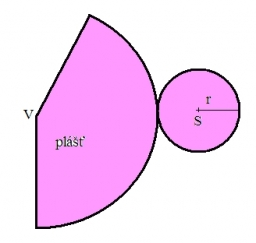# Parameters 28521

The basic parameters of the rotating cone are:
Base radius 5 cm
Cone height 12 cm and cone side 13 cm.
Calculate:
a/volume of the cone
b/cone surface

V =  314.1593 cm3
S =  282.7433 cm2

### Step-by-step explanation:Did you find an error or inaccuracy? Feel free to write us. Thank you!

Tips for related online calculators
Need help calculating sum, simplifying, or multiplying fractions? Try our fraction calculator.
Tip: Our volume units converter will help you convert volume units.
The Pythagorean theorem is the base for the right triangle calculator.

#### Grade of the word problem:

We encourage you to watch this tutorial video on this math problem: